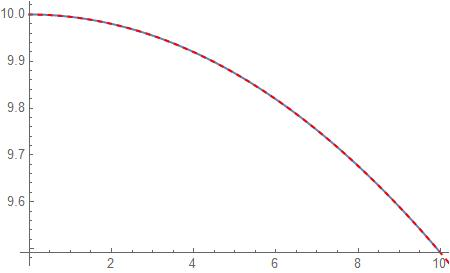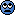# An interesting Nonlinear Differential Equation

• I
TL;DR Summary
I have been thinking about the differential equation for the motion of a free falling object without air resistance (on my own, not as homework), which is in the form ##y^2y''+K=0## with ##K## as a positive constant. I'm looking for advice on a first step to solve this equation.
That's pretty much it. If there is a very basic strategy that I am forgetting from ODEs, please let me know, though I don't recall any strategies for nonlinear second order equations.

I've tried looking up "motion of a free falling object" with various specifications to try to get the solution to this equation, but the sources I find still always assume ##g## does not vary with distance. I have also tried looking up "motion of a charge in a coulomb field" but to no avail.

Mentor
2022 Award
I looked up the solution and it didn't give any hint how to start, it looks awful.

I looked up the solution and it didn't give any hint how to start, it looks awful.
Where does one find the solution? Wolfram Alpha didn't come up with anything.

I could try to reverse engineer it, or in any case check a few things with the solution that I've wanted to look at.

Gold Member
where does the nonlinear term come from if there is no air resistance?

Homework Helper
2022 Award
Summary:: I have been thinking about the differential equation for the motion of a free falling object without air resistance (on my own, not as homework), which is in the form ##y^2y''+K=0## with ##K## as a positive constant. I'm looking for advice on a first step to solve this equation.

That's pretty much it. If there is a very basic strategy that I am forgetting from ODEs, please let me know, though I don't recall any strategies for nonlinear second order equations.

I've tried looking up "motion of a free falling object" with various specifications to try to get the solution to this equation, but the sources I find still always assume ##g## does not vary with distance. I have also tried looking up "motion of a charge in a coulomb field" but to no avail.

This is of the form $y'' = f(y)$ with $f(y) = - K/y^2$, so multiplying by $y'$ and integrating is the first step: $$y' = \pm \sqrt{C + \frac{2K}{y}}.$$ Getting any further requires that you be able to solve $$\int \sqrt{\frac{y}{Cy + 2K}}\,dy = t$$ analytically.

Given your actual question, setting $r = R + y$, where $R$ is the radius of the Earth and $y$ is height above the surface, in $$r'' = \frac{-K}{r^2}$$ will yield $$y'' = - \frac{K}{R^2}\left(1 + \frac{y}{R}\right)^{-2}$$ and on the assumption that $y/R \ll 1$ you can expand the right hand side in binomial series to either O(1) (getting the familiar constant acceleration) or to O(y/R), getting $$y'' = - \frac{K}{R^2}\left(1 - \frac{2y}{R}\right)$$ which is a second-order non-homogenous linear ODE with constant coefficients.

•Isaac0427
Fred Wright
As an alternate approach to @pasmith consider the DE,
$$y^2\ddot{y}=-K$$
and observe,
$$\ddot{y}=\dot{y}\frac{d\dot{y}}{dy}$$
so that
$$\dot{y}\frac{d\dot{y}}{dy}=-\frac{K}{y^2}$$
and thus,
$$\int_{\dot{y}_0}^{\dot{y}}\dot{y}d\dot{y}=-K\int_{y_0}^{y}\frac{dy}{y^2}$$
$$\frac{1}{2}[\dot{y}^2 - \dot{y}_0^2]=K[\frac{1}{y}-\frac{1}{y_0}]$$
where ##y_0## is the initial position and ##\dot{y}_0## is the initial velocity. We have,
$$\frac{dy}{dt}=\sqrt{2}\sqrt{\frac{K}{y} + C}$$
$$C=\frac{1}{2}\dot{y_0}^2-\frac{K}{y_0}$$
$$t=\int_{y_0}^{y}\sqrt{\frac{y}{2K}}\frac{dy}{\sqrt{\frac{Cy}{K}}+1}$$
Make the substitution,
$$u^2=\frac{Cy}{K}$$
to get,
$$t=\frac{\sqrt{2}K}{C^{\frac{3}{2}}}\int_{\sqrt{\frac{Cy_0 }{K}}}^{\sqrt{\frac{Cy }{K}}}\frac{u^2du}{\sqrt{u^2+1}}$$
Integrating by parts we find,
$$t=\frac{K}{\sqrt{2}C^{\frac{3}{2}}}[u\sqrt{u^2+1}-\sinh^{-1}(u)]|_{\sqrt{\frac{Cy_0}{K}}}^{\sqrt{\frac{Cy}{K}}}$$
Clearly ##y(t)## can't be expressed in terms of elementary functions, but you can make a plot of y vs. t by plugging in y values and computing t.

aheight
Here's how I would check it:

In Fred's solution above:
$$(y')^2=2K(1/y-1/y_0)+(y_0')^2$$
I chose the negative root and solved:
$$y'=-\sqrt{2K/y+c},\quad c=(y_0')^2-2K/y_0$$
to obtain:
$$x(c,K,y)=\frac{2 K \tanh ^{-1}\left(\frac{\sqrt{c+\frac{2 K}{y}}}{\sqrt{c}}\right)}{c^{3/2}}-\frac{y \sqrt{c+\frac{2K}{y}}}{c}$$
Next, I solved a test IVP numerically in Mathematica with y(0)=10, y'(0)=0 and K=1 and plotted the solution as the gray trace in the plot below:

Mathematica:
mySol = First@
NDSolve[{y[t]^2 y''[t] + 1 == 0, y == 10, y' == 0},
y, {t, 0, 10}]
theTrace[t_] = Evaluate[Flatten[y[t] /. mySol]];
dPlot = Plot[theTrace[t], {t, 0, 10}]

Then I superimposed a parametric plot of {x(c,K,y),y} over this solution as the red dashed lines to confirm the analytic solution matches the numeric solution:

Mathematica:
myf[c_, k_, y_] = Integrate[-1/Sqrt[2 k/y + c], y]
pp1 = ParametricPlot[{myf[-1/5, 1, y], y}, {y, 9, 10},
AspectRatio -> 1, PlotStyle -> {Dashed, Red}]
Show[{dPlot, pp1}]Last edited:
•Isaac0427
$$L=\frac{1}{2}y'^2-V,\quad V=-\frac{K}{y}$$
1-dimensional motion in Coulomb's field

•epenguin
Homework Helper
Gold Member
Or gravitational - inverse square law? Solutions well knownGravitation can only attract, here attraction or repulsion depend on the sign of K
inverse square law is for force, for the potential just 1/y

Homework Helper
Gold Member
Gravitation can only attract, here attraction or repulsion depend on the sign of K
inverse square law is for force, for the potential just 1/y
Ah, it was late at night, I thought something might be not quite right, note the emoticon. OK he said K positive. But anyone who can do it for K negative can for K positive I'd think.

where does the nonlinear term come from if there is no air resistance?
Force varies with distance from the center of Earth.
and observe,
y¨=y˙dy˙dy
so that
Where does this come from? I may just be missing something obvious here.